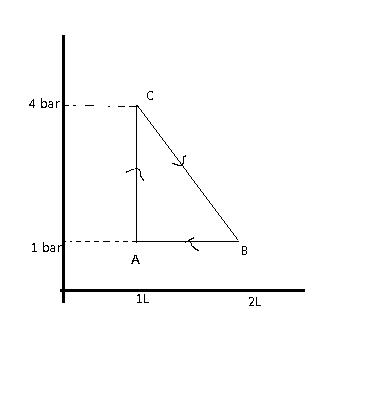Doubts from a Test Paper

1. If the slope " Z (compressibility factor) vs " P " curve is constant ( Slope = Ï€492.6 atm-1) at a particular temperature (300 K) and very high pressure, then calculate the diameter if the molecules?

2.The density of vapour of a substance (X) at 1 atm pressure and 500 K is 0.8 Kg/m3. The vapour effuses through a small hole at a rate 4/5 times slower than oxygen under same condition. What is the compressibility factor (Z) of vapour?

3. Calcute relative rate of effusion of SO2 to CH4, if the mixture obtained by effusing out a mixture with molar ratio nSO2nCH4 = 81 for three effusing steps.

THanks!

•Vivek @ Born this Way ·

4. One mole of a gas is carried through the following reversible process. Find the maximum temperature attained by the gas in K.

Figure:•Vivek @ Born this Way ·

Revive!

•Vivek @ Born this Way ·

Q4. done but first three remain!

•